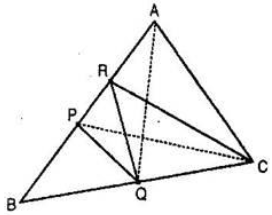Guru

# P and Q are respectively the mid-points of sides AB and BC of a triangle ABC and R is the mid-point of AP, show that: (i) ar (PRQ) = ½ ar (ARC) Q.7(1)

• 0

Please give me the best way for solving the problem of class 9th ncert math of Areas of Parallelograms and Triangles chapter of math of class 9th of exercise 9.4 of question no 7 (1) what is the tricky way for solving this question P and Q are respectively the mid-points of sides AB and BC of a triangle ABC and R is the mid-point of AP, show that: (i) ar (PRQ) = ½ ar (ARC)

Share

1.We know that, median divides the triangle into two triangles of equal area,

PC is the median of ABC.

Ar (ΔBPC) = ar (ΔAPC) ……….(i)

RC is the median of APC.

Ar (ΔARC) = ½ ar (ΔAPC) ……….(ii)

PQ is the median of BPC.

Ar (ΔPQC) = ½ ar (ΔBPC) ……….(iii)

From eq. (i) and (iii), we get,

ar (ΔPQC) = ½ ar (ΔAPC) ……….(iv)

From eq. (ii) and (iv), we get,

ar (ΔPQC) = ar (ΔARC) ……….(v)

P and Q are the mid-points of AB and BC respectively [given]

PQ||AC

and, PA = ½ AC

Since, triangles between same parallel are equal in area, we get,

ar (ΔAPQ) = ar (ΔPQC) ……….(vi)

From eq. (v) and (vi), we obtain,

ar (ΔAPQ) = ar (ΔARC) ……….(vii)

R is the mid-point of AP.

, RQ is the median of APQ.

Ar (ΔPRQ) = ½ ar (ΔAPQ) ……….(viii)

From (vii) and (viii), we get,

ar (ΔPRQ) = ½ ar (ΔARC)

Hence Proved.

• 0